• EXCEL生成正态分布随机数据 如果用Matlab就为简单些，normrnd+normpdf就可实现。但是考虑到excel的普及性，所以采用excel来解决： 1、产生符合正态分布的随机数：输入“＝NORMINV(RAND(),mean,standard_dav)”，...
EXCEL生成正态分布随机数据
如果用Matlab就为简单些，normrnd+normpdf就可实现。但是考虑到excel的普及性，所以采用excel来解决：
1、产生符合正态分布的随机数：输入“＝NORMINV(RAND(),mean,standard_dav)”，mean是均值，standard_dav是标准方差。
2、下拉的方式产生需要数目的随机数，全选，复制，再右键点“选择性粘贴”，选“数值”（这样做的目的是为了将公式形式去掉，不然它会再次产生新的随机数，而你被蒙在鼓里），然后排序。
3、另起一栏，输入“＝NORMDIST(X,mean,stardard_dav,false)”，X是刚才你输入的随机数所在位置，产生概率后，下拉，得到你需要的全部随机数对应的概率，然后就可以作出我们熟悉的正态分布曲线了。

展开全文excel
• 本文首先给出正态分布概率密度函数（The normal distribution probability density function）的公式和标准正态分布概率密度函数的公式，然后通过normpdf( )生成标准正态分布概率密度函数的数据，然后通过plot( )...
本文首先给出正态分布概率密度函数（The normal distribution probability density function）的公式和标准正态分布概率密度函数的公式，然后通过normpdf( )生成标准正态分布概率密度函数的数据，然后通过plot( )绘制标准正态分布概率密度函数的图形。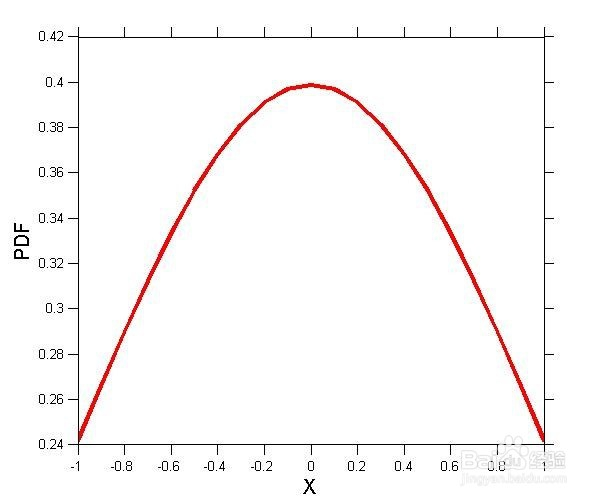工具/原料

MATLAB

normpdf

mean

standard deviation

方法/步骤

第一，正态分布概率密度函数的公式如下图。其中，μ为平均值（mean），σ为标准差（standard deviation）。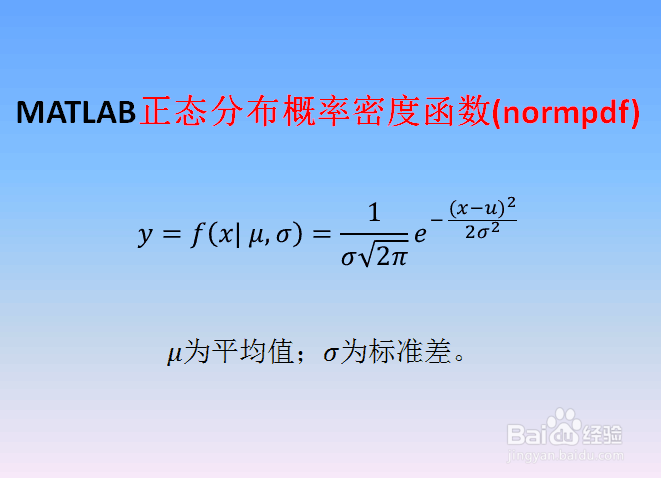第二，当μ=0，σ=1时，第一步中的正态分布为标准正态概率密度函数，如下图。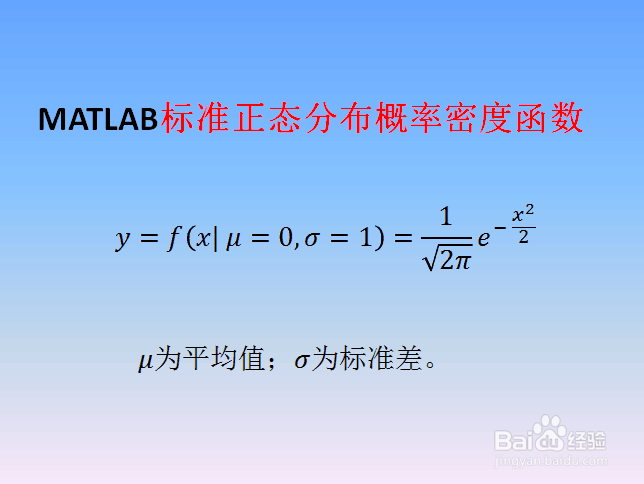第三，启动MATLAB，新建脚本（Ctrl+N），输入如下代码：

close all; clear all; clc

x=-1:.1:1;

norm=normpdf(x,0,1);

figure('Position',[50,50,600,500],'Name','Normal PDF',...

'Color',[1,1,1]);

plot(x,norm,'r-','LineWidth',3)

set(gca,'FontSize',10,'TickDir','out','TickLength',[0.02,0.02])

xlabel('X','FontSize',15);ylabel('PDF','FontSize',15)

其中normpdf(x,0,1)是用来产生μ=0，σ=1的标准正态分布概率密度函数的数据。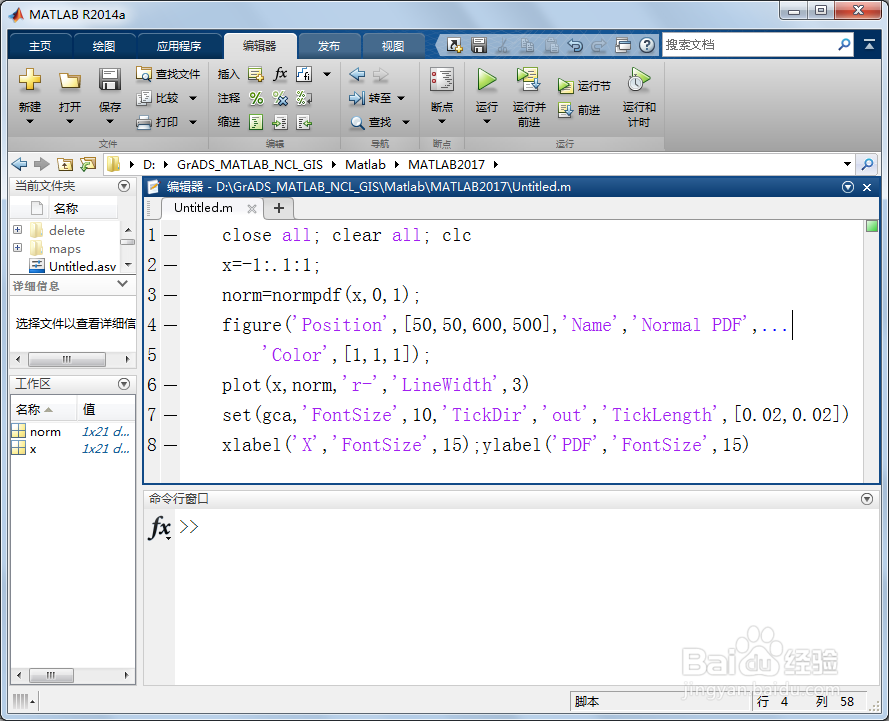第四，保存和运行上述脚本，在工作区（Workspace）得到标准正态分布概率密度函数的数据norm，双击norm可以查看数据具体内容。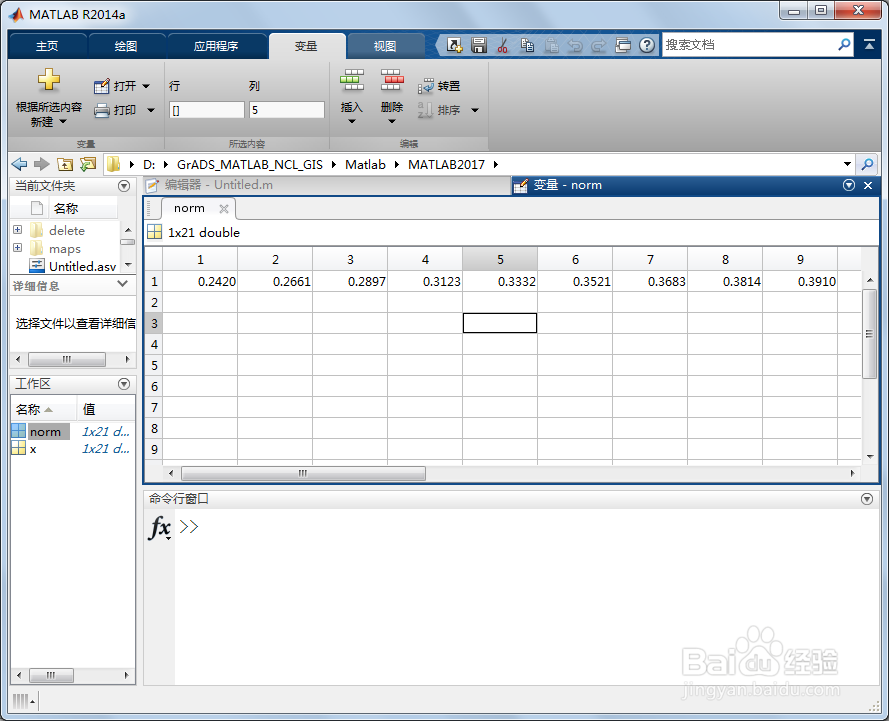第五，同时得到如下μ=0，σ=1的标准正态分布概率密度函数图形。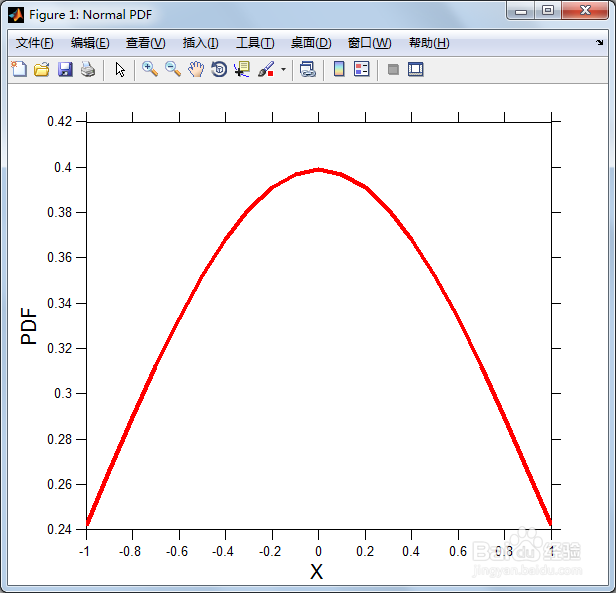展开全文• 详细说明：2.wps ：为作业最终的仿真结果与分析正态分布：.cpp为由C++编写的主程序以获取仿真数据，.txt为存储仿真数据的文档，.m为由matlab根据获取的仿真数据画图的结果，其中1、2、3分别为N(0,1)、N(0,4.26)、N(-...
详细说明：2.wps ：为作业最终的仿真结果与分析正态分布：.cpp为由C++编写的主程序以获取仿真数据，.txt为存储仿真数据的文档，.m为由matlab根据获取的仿真数据画图的结果，其中1、2、3分别为N(0,1)、N(0,4.26)、N(-2.5,1)的情况瑞利分布：.cpp为由C++编写的主程序以获取仿真数据，.txt为存储仿真数据的文档，.m为由matlab根据获取的仿真数据画图的结果，其中1、2、3分别为Ray(1)、Ray(0.36)、Ray(3.4)的情况泊松分布：.cpp为由C++编写的主程序以获取仿真数据，.txt为存储仿真数据的文档，.m为由matlab根据获取的仿真数据画图的结果，其中1、2、3、4分别为Lamda(0.2)、Lamda(1)、Lamda(6)、Lamda(10)的情况0.m为将三或四种情况画到一副图中并进行仿真结果对比的图像-(2.wps:) for the job the final simulation results and analysis of the normal distribution:. Cpp written by C++ main program to obtain the simulation data. Txt document for storing simulation data, m grounds matlab drawing according to the simulation data obtained the results, wherein 1, 2, 3 respectively, for the N (0,1), N (0,4.26), N (-2.5,1), of Rayleigh distribution: CPP grounds C++ prepared by the main program to obtain its simulation data, txt for storage simulation data documents, the. m grounds MatLab drawing the results according to the simulation data obtained, wherein 1,2,3 respectively ray (1), ray (0.36), the case of the ray (3.4) poise the loose distribution: cpp grounds written main program C++ to get the simulation data txt simulation data is stored documents, m grounds matlab based on the results of the simulation data obtained drawing, in which 1, 2, 3, 4, respectively 0.m case of Lamda (0.2), Lamda (1), Lamda (6), Lamda (10) of the three or four painting to a graph,
展开全文• 假设现有一个名为data的1x500的数据，这里我们使用normrnd随机生成一个正态分布数据 data=normrnd(0,5,[1,500]); 可以使用histogram函数对齐分布进行绘制，这里我们把它分成30个bar，可以粗略看出接近正态分布 ...
假设现有一个名为data的1x500的数据，这里我们使用normrnd随机生成一个正态分布的数据

data=normrnd(0,5,[1,500]);

可以使用histogram函数对齐分布进行绘制，这里我们把它分成30个bar，可以粗略看出接近正态分布

histogram(data,30);
xlim([-16,16]);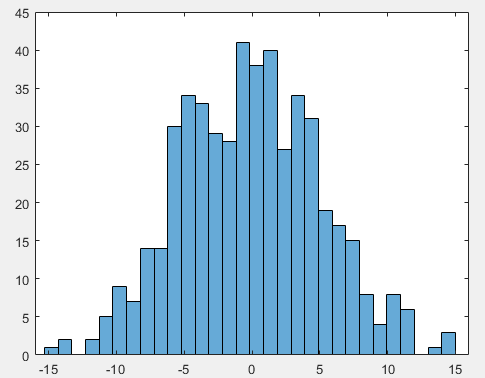如果想画的是频率图而不是频数图可以使用下面的代码

histogram(data,30,'Normalization','probability');

对其进行正态分布拟合，有两种方法可以选用

方法一是直接使用histfit函数,histfit函数会直接生成一个带分布直方图的正态分布拟合

histfit(data,30);
xlim([-16,16]);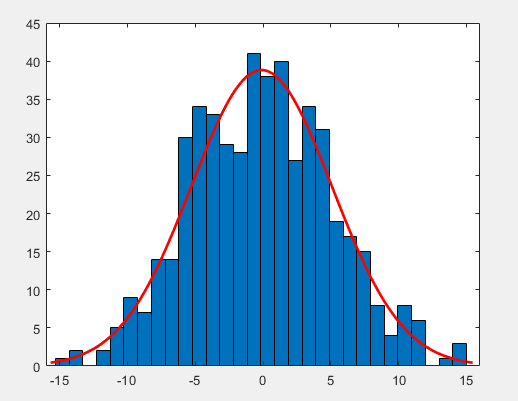方法二则是根据数据的均值和标准差直接拟合,使用函数normpdf生成一个对应的正态分布概率密度函数，乘以数据个数并把它画在上面的图里面，可以看到基本是一致的。这种方法可以单独画曲线，相对于方法一比较灵活。

hold on
x=-16:0.001:16;
fx=normpdf(x,mean(data),std(data));
plot(x,fx*500,'g-');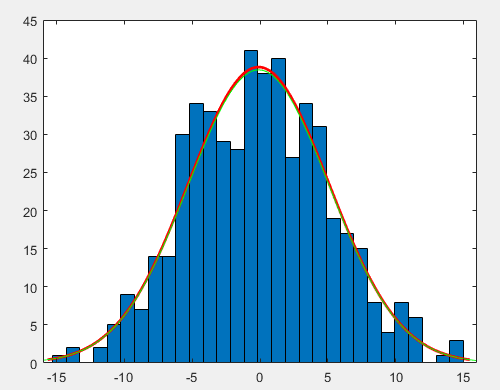展开全文统计模型
• 本篇文章主要讲解在matlab环境下，如何随机生成满足高斯分布的样本点。  众所周知，高斯分布是一种很常见的数据分布，也是很多实际例子中出现最多的一种分布。我们今天就来学习在matlab环境下绘制出随机分布的满足...
• MATLAB的 histfit 函数，可以简单、粗暴的得到数据的柱状图、拟合曲线。 r = normrnd(10,1,100,1); % 生成随机数 figure hf=histfit(r,20); % 20表示将数据均分为20段 pd = fitdist(r,'Normal') % 获得拟合曲线的...
• 本篇文章主要讲解在matlab环境下，如何随机生成满足高斯分布的样本点。众所周知，高斯分布是一种很常见的数据分布，也是很多实际例子中出现最多的一种分布。我们今天就来学习在matlab环境下绘制出随机分布的满足高斯...
• 数据进行正态分布检验 对数据进行正态分布检验，即判断数据是否满足皮尔逊相关系数假设检验的第一个条件。...%生成100*1的随机向量，所有元素是均值为2，标准差为3的正态分布 skewness(x) %偏度 kurtosis(x) %峰数学建模
• 数值分析Matlab二维正态(高斯)分布以及协方差矩阵...代码运行结果生成正态分布实验数据如图：MATLAB代码：mu1 = [0 0]; sigma1 = [4 2 ; 2 4]; r1 = mvnrnd(mu1,sigma1,100); scatter(r1(:,1),r1(:,2),'r.'); hold人工智能 数学 机器学习
• 本篇博客主要讲使用c++标准库生成正态分布数据，然后使用最大似然的方法估计学习参数。并提供c++源码。 一维正态分布满足如下公式： Pr⁡(x)=12πσ2exp⁡[−0.5(x−μ)2/σ2]\operatorname{...机器学习 计算机视觉 C++
• 本篇博客主要讲使用c++标准库生成正态分布数据，然后使用最大似然的方法估计学习参数。并提供c++源码。一维正态分布满足如下公式： n个数据假设独立分布，则联合分布为： 进行极大似然估计，就是选择一组参数，...
• %生成正太随机数据 M=1000; N=1; x=normrnd(1,2,M,N); %生成均匀分布 ...legend(‘正太分布数据’,‘均匀分布数据’); grid on 张德丰编著—MATLAB概率与数据统计分析----【M】机械工业出版社 ...
• matlab生成二维服从高斯分布的数据 2015-12-30 21:31 1263人阅读 评论(0) 收藏 举报  分类： matlab（8）  由于实验需要，需要生成两类模式的数据，同时这两类数据要服从正态分布（高斯...算法 C
• 由于实验需要，需要生成两类模式的数据，同时这两类数据要服从正态分布（高斯分布）。 使用matlab来实现： mu = [2 3]; SIGMA = [1 0; 0 2]; r = mvnrnd(mu,SIGMA,100); plot(r(:,1),r(:,2),'r+'); hold on; ...
• %生成100%的随机向量，每个元素是均值为2，标准差为3的正态分布 skewness(x)%偏度 kertosis(x)%峰度 样本个数越大，检验的结果越好 构造JB统计量 JB=n6[S2+(K−3)24]JB=\frac{n}{6}[S^2+\frac{(K-3)^2}{4}]JB=6n​...python 算法
• 本文目的是介绍如何模拟实际数据中具有特征的时间序列数据。...均匀且正态分布的白噪声和粉红色噪声 可以通过函数rand（均匀分布）和randn（通常为Gaussian分布）生成噪声。这些函数的输入指定所得矩阵的大小。 ...信号处理 生物信号处理
• 正态云模型是基本的云模型，正态分布具有普适性，大量社会和自然科学中定性知识的云的期望曲线都近似服从正态或半正态分布。正态云的数字特征反映了定性概念和定量特性，用期望 Ex( Expected Value) ，熵 En( ...
• 这里我们通过正态分布随机生成两组数据 data1=normrnd(0,5,[1,500]); data2=abs(normrnd(0,5,[1,500])); 他们的分布分别为下图所示： 使用cdfplot函数就可以简单的画出他们的累积分布函数曲线 figure(1) ...统计学
• 网上找到的画热图的方法...以随机生成x和y成正态分布均值为0，方差为10的图为例 X=normrnd(0,10,[100000,1]); Y=normrnd(0,10,[100000,1]); numbins = 50; markersize = 50; marker = '.'; [values, centers] = his
• matlab生成一组正态分布的随机数 a = random('Normal', 3,3, [1, 100]);%均值为3，标准差为3,1行100列 figure,plot(a,'b'),hold on b = (a - mean(a))/std(a,0,2);%std(a,0,2)对于行向量求标准
• randn(n) 生成n×n的满足标准正态分布的随机数 randn(m,n) 生成m×n的满足标准正态分布的...R = normrnd(MU,SIGMA) %返回均值为MU,标准差为SIGMA的正态分布的随机数据,R可以是向量或矩阵. R = normrnd(MU,SIGMA,m)
• 五. 添加图例 （源代码legends.m） ...我们在一幅图像中，同时绘出10个不同均值和方差的正态分布曲线。数据可以由如下代码生成，或直接加载10NormalDistributions.mat % stdVect and meanVect stdVect = [.49,可视化
• ## Matlab常用技巧备忘

千次阅读 2010-01-08 17:29:00
常用命令clear 清空工作区所有变量save filename varname 保存某变量到文件clear xxx 只清空xxx变量clc 清空命令行窗口 数据处理autocorr 求自相关函数crosscorr 求互相关函数randn(m,n) 生成正态分布随机数m行n...session 加密
• 实验 6 Matlab 数值计算 disp' 随机矩阵为 > 实验目地 a disp' 最大值 > 1 掌握数据统计与分析地方法 am disp' 最小值 > 2 掌握数据插值和曲线拟合地方法及其 ai 应用 ... 利用 randn 函数生成符合正态分布地 al disp'
• 原文链接：matlab生成两类服从高斯分布的数据 由于实验需要，需要生成两类模式的数据，同时这两类数据要服从正态分布（高斯分布）。 使用matlab来实现： mu = [2 3]; SIGMA = [1 0; 0 2]; r = mvnrnd(mu,...
• % %Matlab 自带KNN算法函数knnclassify实现 ...%mvnrnd([2 2],eye(2),100)表示随机生成多元正态分布100X2矩阵，每一列以2，2为均值，eye(2)为协方差 %200个样本数据前100标记为标签1，后100个标记为标签2
• Tutorial 6 实验报告 实验名称Matlab数值计算 实验目的 掌握数据统计与分析的方法 掌握数据插值和曲线拟合的方法及其应用 掌握多项式的常用运算 实验内容 利用randn函数生成符合正态分布的105随机矩阵A进行如下操作 ...# matlab生成正态分布数据matlab 订阅